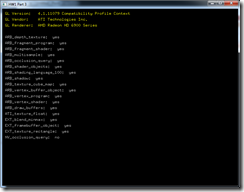## 3. Transformations I: Linear Transformations (vector, matrix)

3. Transformations I: Linear Transformations. Multiple Transformations (2/14) … vectors & linear transformations (4×4 matrices)

Vector is length & direction, can be represented as 2D (dx, dy) or 3D (dx, dy, dz).  Add vectors adds components (visually attach tail to head).  Subtract vector just add the negative (visually flip direction of minus vector).  To compute the length, use pythagorean theorem.  Unit vector is vector length 1, compute by dividing the vector by its length.

Dot product A dot B = AxBx + AyBy + AzBz = cos(theta) * ||A|| * ||B||.  Theta is the angle between A and B.  ||A|| is magnitude of A.  For unit vectors, A dot B = cos(theta).  Because for unit vectors, ||A|| is 1 and ||B|| is 1.  Dot product can tell you which side of a surface something is on.  Example take the dot product of the Normal vector (to a plane) and the View vector (to the camera); the sign (greater than or less than zero) tells you if the angle is less than or greater than 90 degrees

## 2. Light, Illumination & Reflectance

Notes on lecture, 2. Illumination. What is light? The BRDF. Vectors and dot products. The Phong BRDF model (2/7)

## Homework 1

Homework 1 was just to compile and run their sample project.  I opened the sln file with VS 2010, and let it convert from VS 2005 to VS 2010.  Other than that, it just compiled and ran without any effort on my part.  It just prints GL version, GL vendor, GL Renderer (my GPU), and "yes" or "no" for whether my GPU supports some specific OpenGL extensions.The OpenGL version used by the code samples (and homeworks) is the one thing I

## 1. Intro: Course, GPU, Computer Grfx

1. Introduction. Course overview. What is computer graphics? GPU overview (1/31)

Harvard Extensions School, CSCI E-234 of Spring 2007.  OpenGL / GLSL, computer graphics.  Offline rendering vs. Interactive rendering, rise of GPU graphics.  App -> API -> driver -> GPU (driver sends commands to GPU).  Graphics Pipeline: App -> Cmd -> GPU, where GPU was: Geometry -> Rasterization -> Fragment -> Display.  Move from Fixed Function to Programmable Pipeline: VS -> RS -> FS (ogl FS = dx PS).  Vertex Shaders, Pixel Shaders => grfx more like Ray Tracing.  2011 updates…  dx10 added GS, dx11 added T* & CS.  Dx11 Pipeline: IA -> VS -> (HS -> TS -> DS) -> GS -> RS -> PS -> OM.  Or GPGPU such as OpenCL and DirectCompute (dx CS).  Modern API (software) has Unified Shader Model.  Modern GPU (hardware) has Unified Shader Architecture.

Topics: interactive 3d grfx, OpenGL grfx pipeline, special effects (bump maps, shadows, etc).  Ray tracing (off-line render).  Fundamentals (light, color, camera models, bezier splines, etc).  Books: OpenGL Programming Guide 4th Ed (red book) (isbn 0023548568), Computer Graphics Using OpenGL by Francis s. Hill Jr (isbn 0201604582), others suggested.  Weekly class 2 hours, weekly sections 2 hours (sadly, these weren

## Harvard Intro to Computer Graphics

This section will be for my notes on CS E-234 of Spring 2007 (January 31, 2007 through May 16, 2007) – the 13 videos (~2 hours each) are from iTunes U.  My plan is to watch the lectures slowly-over-time in 45 min sessions while jogging on an elliptical.  Then take some paper notes, maybe do some extra reading, maybe write some code, and put some notes here.  This is pretty basic (fundamental) stuff, so I